# An Enhanced Secure, Robust and Efficient Crypto Scheme for Ensuring Data Privacy in Public Cloud Using Obfuscation & Encryption

An Enhanced Secure, Robust and Efficient Crypto Scheme for Ensuring Data Privacy in Public Cloud Using Obfuscation & Encryption
Department of CSE, Vignan’s Foundation for ScienceTechnology and Research, Vadlamudi 522213, AP, India

Department of CSE, Vignan’s Foundation for ScienceTechnology and Research, Vadlamudi 522213, AP, India

Corresponding Author Email:
dy_cse@vignan.ac.in
Page:
603-609
|
DOI:
https://doi.org/10.18280/isi.240607
9 August 2019
|
Accepted:
20 October 2019
|
Published:
31 December 2019
| Citation

OPEN ACCESS

Abstract:

Cloud has been emerging, popular and very demanding technology now a day. Cloud has got wide popularity with its sophisticated features. The primary features of cloud include internet access, more storage, easy setup, automatic updates, and low cost and resource provisioning based on “pay as you go” policy. In spite of advantages, security is considered to be more important and drew the attention of many researchers because it is not guaranteed in an open cloud. The data storage is becoming an indispensable measurement in cloud and most of the times cloud does not guarantee that data that has been stored is secured from illegitimate access. Many researchers are working to ensure data security in the cloud but unfortunately they do not provide adequate security to data. This paper is aiming to propose a secure hybrid scheme with obfuscation and cryptography to ensure the privacy of data shared in public cloud. Experimental results show that the proposed scheme yields good results.

Keywords:

cloud computing, privacy, obfuscation, cryptography

1. Introduction

Cloud Computing is an emerging technology, and every organization wants to adopt this technology these days. It is cost-effective and simple to use. The user pays as he uses cloud resources . Significant beneficiaries are smaller enterprises and individual professionals who develop applications without having huge investments. It is affordable as pays for what he uses. This technology mainly works with three modules. One is cloud service provider , who places the data or resources into the cloud. The second module is cloud service consumer who accesses the resources necessary from cloud. And the third module is a web interface through which cloud users can place the resources in the cloud or access the services of the cloud . These services can be categorized as Software as a Service (SaaS), Infrastructure as a Service (IaaS), Application as a Service (AaaS), and Platform as a Service (PaaS). These services will be deployed in three models, such as through Public Cloud, Private Cloud, and Hybrid Cloud . The cloud technology has the following features: pay-as-you-go, flexibility, auto-scaling, elasticity, etc. According to pay-as-you-go, the billing process is done based on the amount of utilization of the resources. This feature is playing vital role in the rise of cloud computing technology .

In spite of having such greater features, it comes up with security issues . The security issues are related to information security , network security, infrastructure security, confidentiality, availability [8, 9]. The major research work focused on providing security to data in the cloud from being accessed by illegitimate users . This is known as information security or data security which is the subject of our work . Providing protection to data while it is moving in the network from being attacked by the third party is known as network security. Information that is stored in cloud, should be kept confidential according to the requirements of the cloud user. Cloud computing still not guaranteed information security [12, 13]. Because of such problems in security, this technology is far away from the banking field. Data that is stored/shared in the cloud typically include customer transaction information, customer preferences/feedback/survey feedback, and patients' health information, etc. Hence, applications in cloud computing research typically require effective techniques/algorithms to preserve the privacy of cloud user's confidential information. These techniques/algorithms should work effectively by properly processing, testing and validating the large amounts of data. Real life data that contains sensitive information is more desirable for verifying the performance of these algorithms and techniques.

Effective techniques and algorithms must be introduced to preserve the privacy of such customer transaction information before it is made public or otherwise disseminated . Some techniques work in way that does not allow the illegitimate user to capture the confidential information of authorized users but retains the readability of document . Other techniques include cryptography, which converts the data into some other form that can't be read and understand by anyone unless it is converted into its original form [16, 17]. In the first case, though it does not provides actual information, there is still a possibility of data breach since it allows data readability. In the second case, though it does not enable the readability, there is a possibility that third person can decrypt the data and use it. Hence, techniques should be designed such that no sensitive information is exposed to the illegitimate user, but enough information should be retained for end user to perform other analytical and processing applications on the data [18-20].

In the proposed work was designed and implemented a scheme for preserving the privacy of customer transaction information. Ours protects the data at two levels by preserving privacy at each level. At the first level, it replaces the original information with dummy information, allowsthe readability of the document and it is possible to recreate the original document. Preserving readability of document has few advantages: (i) when third party (illegitimate user) access get access to document, he can't conclude which data is original and which is replaced, (ii) since enough information available, we can perform analytical and processing application on document. At the second level it converts the sensitive information into cipher form to prevent readability. It primarily works on numerical data of customer transaction information and experimental results show that it yields effective results.

The overview of this paper is as follows. Section II describes the related works on ensuring data security. Section III explains the working of the proposed algorithm. Section IV provides experiments with proposed algorithm with sample data. Section V ends in the conclusion.

2. Related Works

Yang and Jia  proposed an efficient and secure dynamic auditing protocol for data storage in cloud computing to protect the confidentiality of data. The cryptic method with binary asset properties of bilinear parsing without using masking technology. Thus our multi-cloud batch auditing protocol does not require any additional organizer. Our batch auditing protocol can also support batch auditing for multiple owners. The advantages are it protects the data privacy against the auditor by combining the cryptography method with the linearity property of bilinear paring, rather than using the masking technique.

Bhadaurya and Sanyal survey on security issues on cloud computing and related relief techniques which proposed cloud classification of continuous development of cloud computing . The most commonly used public cloud, private cloud, hybrid cloud, and community cloud are also used by IaaS mainly used by the lowest layer the PaaS is used in the layer and the SaaS uses the highest layer. Data in transit, data-at-rest, data lineage, data remission, data provinces and data infringement are mainly used where security vulnerability such as copying, transmitting or stealing sensitive protected or confidential data infringes data infringement or unauthorized use. The benefits of Cloud computing is the computing world is revolutionary it is likely to have different security threats to level threats applied from network-level threats. To keep the cloud safe these security threats must be regulated. In addition cloud service providers have met all SLAs and should not have human defects on their part and should be reduced to smooth performance.

Arul Oli and Arockiam here proposed an obfuscation technique to protect numerical data in cloud storage . When the user wants to encrypt the inconsistent numerical data with confusion the proposed technology is flexible and compatible. This method is a symmetric cryptosystem. There are two keys used in this proposed algorithm for encryption and decryption. And two keys are integer values. With these two keys opacity of numerical data is possible through the proposed ARO_Obfus_CT to preserve data in the public cloud.

Arul Oli and Arockiam proposed an AO_ARO_EncObfus_CT is a symmetric cryptosystem Combined with encryption and obfuscation encrypting process and neglecting non-numeric and numeric data respectively . The procedure is performed simultaneously. When the user wants to hide all the data numeric and non-numeric type then the user must use the proposed algorithm. After selecting this CT the user will inform the CSP and select the technique Process. During the process the technique stimulates request ASP for AO_Enc_CT and ARO_Obfus_CT Press KMaaS to generate keys for CT. KMaaS produce the keys required for encryption of non-numeric data the plain text given.

Authors Dharani  and others have proposed that the creative cryptographic algorithms and digital signature methods are credible and efficient to provide more security of the user's data in the cloud. The need for cloud computing analyzed data security and added mathematical modeling on the basis of these requirements.

3. Proposed Scheme

The proposed scheme aims at securing the customer transaction information that has been shared through the public cloud. It preserves the privacy of the customer's sensitive data. It primarily works on numerical data so that processing time could be reduced to a significant level when compared with existing techniques that work on entire document. It secures data at two levels: At first level it replaces the original information with dummy information, allows readability of the document and it is possible to generate the original document. Preserving readability of document has few advantages: (i) when third party (illegitimate user) get access to document, he can't conclude which data is original and which is replaced, (ii) since enough information available, we can perform analytical and processing application on document. At the second level it converts the sensitive information into cipher form to prevent readability . Figure 1 shows the detailed structure of proposed scheme.

## 1.pngFigure 1. Overview of the proposed scheme

3.1 Modules

The proposed algorithm has four modules, Obfuscator, Cryptanalysis scheme module, Request processing, and Secure storage.

Obfuscator: Modules of the proposed scheme is shown in Figure 2. This module performs the obfuscation and de-obfuscation operations on the input document. Whenever a customer wants to store his confidential information in cloud, the obfuscator module obfuscates the document and sends it to cryptanalysis module. And de-obfuscate the document, whenever customer wants to use it. It obfuscate the sensitive information (numerical data) in the document that is in either structured or unstructured format. This module attempts to create the obfuscated document and retain the readability of the document. It obfuscate the document with false values in a manner that is still possible and easy to recreate the original document from it. The detailed workflow of the module is described in Figure 3 & Figure 4.

Cryptanalysis: This module performs the encryption modules and decryption operations on the document. Upon receiving the obfuscated document from the obfuscator module, it performs the encryption operation on numerical data alone.  Then the encrypted document will be stored in cloud. Whenever customer wants to use it, it first decrypts the document and sends it to obfuscator module which then performs the de-obfuscation to recreate the original document.  The encryption and decryption process takes less processing time when compared with existing techniques that work on whole document.

Request processing: This module takes the responsibility of sending and receiving requests to cloud users.

Secure storage: Used to store cloud resources securely.

## 2.pngFigure 2. Modules of the proposed scheme

## 3.png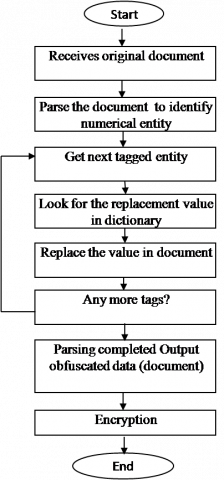Figure 3. Transforming the original document into cipher

## 4.png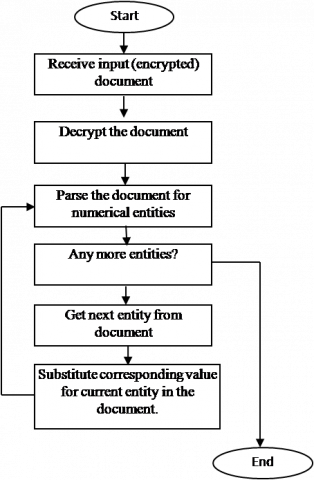Figure 4. Transforming the cipher document into original

3.2 Detailed workflow of modules

The below Figure 5 & Figure 6 show the detailed flow of modules that works to create a cipher document and recreates the original document from the cipher.

## 5.pngFigure 5. Process of generating value table

In Figure 5, the Obfuscator module after receiving the document (D) from the customer a.0s an input, parses the document to identify the numeric entities in each tuple, which is found to be more sensitive in customer transaction information (example: account number, pin number, one-time passwords, etc.).

Input $\rightarrow$ document $(D)$ with 'n'  no. of tuples $(t), D=$ $\{t 1, t 2, \ldots t n\}$

Identify a numeric entity set $(E)$ in each tuple. $E=$ $\{e 1, e 2 \ldots e n\}$

$f(E)=\sum_{t=1}^{n} \sum_{l=1}^{l-1} x=n \forall x \in\{0,1,2, \ldots .9\}$    (1)

It identifies entities in each tuple using above Eq. (1), and generates obfuscated value for each entity. Then it replaces the value with obfuscated value using Eq. (2). The generation of obfuscated values for each entity is done as follows.

Step 1: User request $R=\{M a c I D+I P+\text {Time stamp }\}$

Step 2: Service provider generates values table from user request $V T=\{0,1,2 \ldots .9\}$

Step 3: Assign index $I=\{0,1,2, \ldots .9\}$

Step 4: For each digit in the entity, look for index equal to the digit and replace with its corresponding value.

If there is an entity "3719" in the document then the corresponding obfuscated value would be "8427".

## 6.pngFigure 6. Process of generating dummy value

Look for the next entity, if it exist it repeats the same process else it outputs the obfuscated document (D') and sends it to the cryptanalysis module, which further encrypts and decrypts the document for more security.

$f(O)=\sum_{t=1}^{n} \sum_{l=1}^{l-1} \quad \forall \mathrm{x} \rightarrow \mathrm{x}^{\prime},$ where $x^{\prime}=\left\{e^{\prime} 1, e^{\prime} 2, \ldots e^{\prime} n\right\}$   (2)

Output $\rightarrow$ Obfuscated document $\left(D^{\prime}\right)$ with $^{\prime} n^{\prime}$ no. of tuples $\left(t^{\prime}\right)$ $D^{\prime}=\left\{t^{\prime} 1, t^{\prime} 2 \ldots . t^{\prime} n\right\}$

In Figure 6, when it receives the obfuscated document (D') as input from the cryptanalysis module, it recreates the original document (D) by substituting the obfuscated entities with original entities (de-obfuscation) in value table using Eq. (3). The flow is as follows. After receiving document, it parses the document for entities, replaces it with its original value in the value table. Look for next entity, if exists repeat the same process, else completes the de-obfuscation process and sends the original document (D) to customer. Example of obfuscation/de-obfuscation of a given document is given in Figure 7.

## 7.pngFigure 7. Example of obfuscation/deobfuscation of a given document

Input $\rightarrow$ Obfuscated document $\left(D^{\prime}\right)$ with 'n' no. of tuples ( $t^{\prime}$ ). $D^{\prime}=\left\{t^{\prime} 1, t^{\prime} 2 \ldots . t^{\prime} n\right\}$

Identify the mumeric entity set $\left(E^{\prime}\right)$ in each tuple. $E^{\prime}=$ $\left\{e^{\prime} 1, e^{\prime} 2 \ldots e^{\prime} n\right\}$

$f\left(O^{\prime}\right)=\sum_{t=1}^{n} \sum_{l=1}^{l-1} \quad \forall x^{\prime} \rightarrow x,$ where $x=\{e 1, e 2, \ldots e n\}$   (3)

The cryptanalysis module receives the obfuscated document as input and identifies the numeric entities in each tuple using Eq. (1). It generates a random key1 (K1) and multiplies the K with all entities identified using Eq. (4).

$f(K E)=\sum_{e=1}^{n} \mathrm{e} * \mathrm{K}_{1}, \forall \mathrm{K} \in \mathrm{N}$        (4)

Next, it computes the square value (SQi) of all multiplied values (KEs) using Eq. (5).

$f(S Q)=\sum_{k e=1}^{n} K E * K E$        (5)

Then it generates random key K2 and rotates the square values from right to left with the number of K2 times. And K2 is incremented by one for consecutive values in SQ(i), K+i, where i=1,2,3,…n. And find the modulus of each rotated square value using Eq. 6. Finally, compute the ASCII value of each modulus which will be in the form of cipher. Figure 8 shows the example of encrypted form of given document.

$f(M o d)=\sum_{r s q=1}^{n} R S Q \% 256$       (6)

For the decryption, it performs the steps in reverse order to convert the entities from cipher form to plain form. The below algorithm shows how information scrambled/replaced with dummy information, and encryption & decryption process.

## 8.jpgFigure 8. Example of the encrypted document

3.3 Proposed algorithm

1. Input: Document (D) with 'n' no. of tuples (t).

$D=\{t 1, t 2, \ldots t n\}$

2. Output: Document (De) with ciphertext CT

3. Start

//Input the original document (D)

4. Input $\rightarrow D, D=\left\{t_{1}, t_{2}, \ldots t_{n}\right\}$

// Identify numeric entity set (E) in each tuple.

$E=\{e 1, e 2 \ldots e n\}$

5. $f(E)=\sum_{t=1}^{n} \sum_{l=1}^{l-1} x=n \forall x \in\{0,1,2, \ldots 9\}$

// Obfuscate the document $\left(D^{\prime}\right), D^{\prime}=\left\{t^{\prime} 1, t^{\prime} 2 \ldots . . t^{\prime} n\right\}$.

6.  $f(O)=\sum_{t=1}^{n} \sum_{l=1}^{l-1} \quad \forall x \rightarrow x^{\prime}$,

where $x^{\prime}=\left\{e^{\prime} 1, e^{\prime} 2, \ldots e^{\prime} n\right\}$

// Output the obfuscated document (D')

7. Output $\rightarrow D^{\prime}, D^{\prime}=\left\{t^{\prime} 1, t^{\prime} 2 \ldots . t^{\prime} n\right\}$

8. Generate a key $K 1,$ where $K 1 \in N=\{0,1,2 \ldots\}$

//Multiply K1 with all numeric entities

9. $f(K E)=\sum_{e=1}^{n} \mathrm{e} * \mathrm{K}_{1}, \forall \mathrm{K} \in \mathrm{N}$

// Compute the square value of $K E$

10. $f(S Q)=\sum_{k e=1}^{n} K E * K E$

11. Generate the another key K2, where $K2 \in N$

$=\{0,1,2 \ldots\}$

//Rotate the SQ from left to right K2 times by incrementing $K 2$ by 1 each time

12. $f(R T)=\operatorname{Rotate}(S Q(i), K 2+i)$

where $i=1,2 \ldots<=N$

// Compute the modulus of $f(R T)$ by dividing with 256

13. $f(M o d)=\sum_{r s q=1}^{n} R S Q \% 256$

// Convert the f(Mod) into ASCII values

14. $C T(i)=A S C I I(f(M o d))$

15. $C T=$ cipher Text

16. End

4. Results & Discussions

The proposed scheme is implemented on CloudSim and performance of the same is verified with existing techniques in terms of time taken for both obfuscation/encryption and decryption/deobfuscation. The experimental results show that the security level of proposed scheme is higher than existing techniques.

Table 1. Processing time of existing and proposed techniques for encryption and obfuscation

 File Size (MB) AO_ARO_EncObfus_CT (MilliSec) Proposed (MilliSec) 1 MB 225 221 2 MB 452 408 3 MB 641 632 4 MB 873 820 5 MB 989 973 10 MB 2239 2212 15 MB 3374 3256

Table 1 contains the processing time of existing and proposed schemes for encryption & obfuscation of a file in various sizes. Figure 9 shows that the proposed scheme takes less time for both obfuscation and encryption when compared with the existing scheme.

## 9.png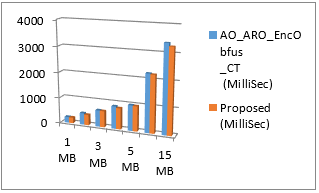Figure 9. Comparison between existing and proposed techniques processing time for encryption and obfuscation

Table 2. Processing time of existing and proposed techniques for decryption and de-obfuscation

 File Size (MB) AO_ARO_EncObfus_CT (MilliSec) Proposed (MilliSec) 1 MB 232 224 2 MB 434 412 3 MB 623 602 4 MB 840 824 5 MB 1063 1003 10 MB 2212 2201 15 MB 3339 3256

## 10.png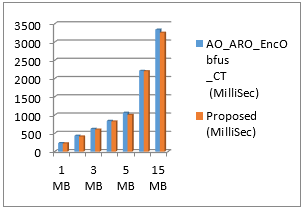Figure 10. Comparison between existing and proposed techniques processing time for decryption and de-obfuscation

Table 3. Level of security offered by existing and proposed schemes

 Crypto Techniques Security level AO_ARO_EncObfus_CT 93 Prposed 96

Table 2 contains the processing time of existing and proposed schemes for decryption & de-obfuscation of a file in various sizes. Figure 10 shows that the proposed scheme takes less time for both obfuscation and encryption when compared with the existing scheme.

Table 3 contains level of security offered by existing and proposed schemes. Figure 11 shows that the security level offered by the proposed scheme is much higher than the existing scheme.

## 11.png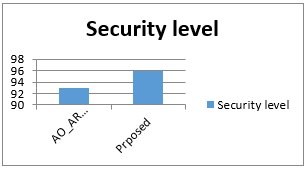Figure 11. Comparison between security levels of existing and proposed schemes

5. Conclusion

This work is aimed to propose an efficient algorithm with obfuscation and cryptography for structured data, which preserve the confidentiality of data stored in cloud at two levels. At the first level, the algorithm obfuscates the document, and at the second level obfuscated document is encrypted using traditional RSA algorithm for better security.The proposed scheme is implemented on CloudSim and performance of the same is verified with existing techniques in terms of time taken for both obfuscation/encryption and decryption/deobfuscation. The experimental results show that security level of proposed scheme is higher than existing techniques.

References

 Khorshed, M.T., Ali, A.B.M.S., Wasimi, S.A. (2012). A survey on gaps, threat remediation challenges and some thoughts for proactive attack detection in cloud computing. Future Generation Computer Systems, 28(6): 833-851. https://doi.org/10.1016/j.future.2012.01.006

 Calheiros, R.N., Vecchiola, C., Karunamoorthy, D., Buyya, R. (2012). The aneka platform and QoS-driven resource provisioning for elastic applications on hybrid clouds. uture Generation Computer Systems, 28(6): 861-870. https://doi.org/10.1016/j.future.2011.07.005

 Halabi, T., Bellaiche, M. (2018). A broker-based framework for standardization and management of Cloud Security-SLAs. Computers & Security, 75: 59-71. https://doi.org/10.1016/j.cose.2018.01.019

 Chauhan, S.S., Pilli, E.S., Joshi, R.C., Singh, G., Govil, M.C. (2018). Brokering in interconnected cloud computing environments: A survey. Journal of Parallel and Distributed Computing, 133: 193-209. https://doi.org/10.1016/j.jpdc.2018.08.001

 Ferrer, A.J. (2016). Inter-cloud research: Vision for 2020. Procedia Computer Science, 97: 140-143. https://doi.org/10.1016/j.procs.2016.08.292

 Asad, S., Fatima, M., Saeed, A., Raza, I. (2017). Multilevel classification of security concerns in cloud computing. Applied Computing and Informatics, 13(1): 57-65. https://doi.org/10.1016/j.aci.2016.03.001

 Sun, Y., Zhang, J., Xiong, Y., Zhu, G. (2014). Data security and privacy in cloud computing. International Journal of Distributed Sensor Networks, 10(7). https://doi.org/10.1155/2014/190903

 Rao, B.T. (2016). A study on data storage security issues in cloud computing. Procedia Computer Science, 92: 128-135. https://doi.org/10.1016/j.procs.2016.07.335

 Wang, R. (2017). Research on data security technology based on cloud storage. Procedia Engineering, 174: 1340-1355. https://doi.org/10.1016/j.proeng.2017.01.286

 Hidayah, N., Rahman, A., Choo, K.R. (2014). A survey of information security incident handling in the cloud. Computers & Security, 49: 45-69. https://doi.org/10.1016/j.cose.2014.11.006

 Singh, S., Jeong, Y., Hyuk, J. (2016). A survey on cloud computing security: Issues, threats, and solutions. Journal of Network and Computer Applications, 75: 200-222. https://doi.org/10.1016/j.jnca.2016.09.002

 Bhadauria, R., Sanyal, S. (2012). Survey on security issues in cloud computing and associated mitigation techniques. International Journal of Computer Applications. https://doi.org/10.5120/7292-0578

 Usman, M., Ahmad, M., He, X. (2017). Cryptography-based secure data storage and sharing using HEVC and public clouds. Information Sciences, 387: 90-102. https://doi.org/10.1016/j.ins.2016.08.059

 Gowthami, G., Yakobu, D., Gnaneswara Rao, N., Amudhavel, J. (2017). An analysis of cloud data security issues and mechanisms. International Journal of Pure and Applied  Mathematics, 116(6): 141-147.

 Hashemzade, B., Maroosi, A. (2018). Hybrid obfuscation using signals and encryption. Journal of Computer Networks and Communications, 2018: 1-6. https://doi.org/10.1155/2018/6873807

 Jorstad N.D., Smith, L.T. (1997). Cryptographic algorithm metrics. Proceedings of 20th NISSC-97, pp. 1-38.

 Ghosh, A., Saha, A. (2013). A numerical methos based encryption. ACER 2013, pp. 149-157. https://doi.org/10.5121/csit.2013.3214

  Horvath, M., Butty, L. (2018). The Birth of Cryptographic Obfuscation – A Survey. The National Research, Development and Innovation Office – NKFIH of Hungary Under Grant Contract no. 116675 (K), pp. 1-59.

 Murthy, T.S., Gopalan, N.P., Yakobu, D. (2019). An Efficient un-realization algorithm for privacy preserving decision tree learning using McDiarmid’s bound. International Journal of Innovative Technology and Exploring Engineering (IJITEE), 8(4S2): 499-502.

 Usman, M., Jan, M.A., He, X.J. (2017). Cryptography-based secure data storage and sharing using hevc and public clouds. Journal of Information Sciences, 387: 90-102. https://doi.org/10.1016/j.ins.2016.08.059

 Yang, K., Jia, X. (2013). An efficient and secure dynamic auditing protocol for data storage in cloud computing. IEEE Trans. Parallel Distrib. Syst., 24(9): 1717-1726. https://doi.org/10.1109/TPDS.2012.278

 Bhadauria, R., Sanyal, S. (2012). Survey on security issues in cloud computing and associated mitigation techniques. International Journal Computer Application, 47(18): 47-66. https://doi.org/10.5120/7292-0578

 Arul Oli, S., Arockiam, L. (2016). Confidentiality technique for data stored in public cloud storage. International Journal Engineering Research & Technology, 5(2): 169-174. https://doi.org/10.17577/ijertv5is020028

 Dharini, A., Devi, R.M.S., Chandrasekar, I. (2014). Data security for cloud computing using RSA with magic square algorithm. International Journal of Innovation and Scientific Research, 11(2): 439-444.

 Balakrishnan, S.V., Ananthanarayanan, R., Datta, S. (2010). Data obfuscation of text data using entity detection and replacement. United States Patent, 2(12): 1-5.